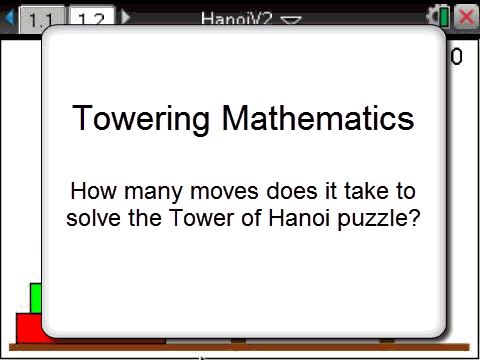# Activities

•30 Minutes

4.5

## Tower of Hanoi#### Activity Overview

Students explore the relationship between the number of discs and moves required to solve the classic “Tower of Hanoi” problem. The TI-Nspire file contains an interactive model produced by Andy Kemp.  The interactive model allows you to change the number of disks and automatically record the total number of moves allowing students to focus on solving the problem and identifying patterns. The activity also includes a degree of scaffolding to help student identify and understand the nature of the geometric recursive relationship between the number of disks and the number of moves.

#### Objectives

• Generation of a geometric recursion using a spreadsheet
• Use the calculator to generate the formula and graph a Geometric Sequence
• Use accurate mathematical notation.

• Geometric
• Recursion
• Term